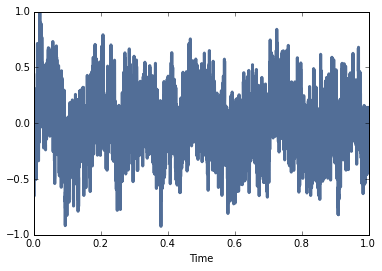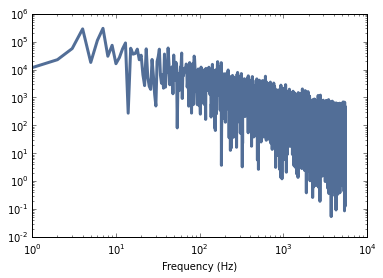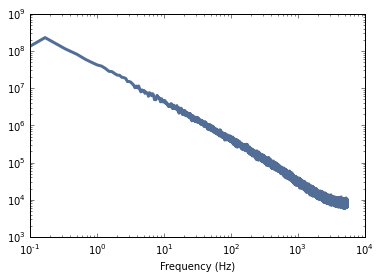# Generating pink noise

January 20, 20161 comment

In one of his most famous columns for Scientific American, Martin Gardner wrote about pink noise and its relation to fractal music.  The article was based on a 1978 paper by Voss and Clarke, which presents, among other things, a simple algorithm for generating pink noise, also known as 1/f noise.

The fundamental idea of the algorithm is to add up several sequences of uniform random numbers that get updated at different rates. The first source gets updated at every time step; the second source every other time step, the third source ever fourth step, and so on.

In the original algorithm, the updates are evenly spaced.  In 1999, in a discussion on the Music-DSP mailing list, McCartney suggested a way to stagger the updates so that only one sequence is updated at each time step:

Here's how to improve the Gardner pink noise generator. Gardner adds up several uniform random number generators that are evaluated in octave time intervals. The pattern is as follows:

x x x x x x x x x x x x x x x x x
x   x   x   x   x   x   x   x   x
x       x       x       x       x
x               x               x
x                               x


Now the problem with the above is that on some samples you have to add up a lot more random values than others. This can also cause large discontinuities in the wave when lots of the values change at once.  By rearranging the order that the random generators change the load can be made even, the operation can be made more efficient and the deviation from any one sample to another is bounded by a constant value every sample. Here's the pattern, a tree structure:

x x x x x x x x x x x x x x x x
x   x   x   x   x   x   x   x
x       x       x       x
x               x
x


Only one of the generators changes each sample. This makes the load constant. It also means that you can update the sum by subtracting the previous value of a generator and adding the new value, instead of summing them all together. You can determine which random number needs to be changed each sample by incrementing a counter and counting the trailing zeroes in the word. The number of trailing zeroes is the index of the random number to change. Number of trailing zeroes can be determined very quickly on a machine with a count leading zeroes instruction.

Remainder of excercise left to the reader.

Then in 2006, Trammell proposed a stochastic version where the updates occur at random intervals instead of equally spaced intervals.

The nice thing about all of these algorithms is that they are easy and efficient to implement incrementally; that is, they can generate one value at a time with only a few operations per value.

However, the properties that make them good for incremental generation make them less good for batch generation, especially in Python and NumPy.  One of the keys to making NumPy computations fast is to express algorithms in terms of array operations.  Incremental algorithms often don't fit the pattern.

So I took it as a challenge to implement the stochastic Voss-McCartney algorithm in Python with reasonable efficiency.  Here's what I came up with:

def voss(nrows, ncols=16):
"""Generates pink noise using the Voss-McCartney algorithm.

nrows: number of values to generate
rcols: number of random sources to add

returns: NumPy array
"""
array = np.empty((nrows, ncols))
array.fill(np.nan)
array[0, :] = np.random.random(ncols)
array[:, 0] = np.random.random(nrows)

# the total number of changes is nrows
n = nrows
cols = np.random.geometric(0.5, n)
cols[cols >= ncols] = 0
rows = np.random.randint(nrows, size=n)
array[rows, cols] = np.random.random(n)

df = pd.DataFrame(array)
df.fillna(method='ffill', axis=0, inplace=True)
total = df.sum(axis=1)



In this function NumPy is imported as np and Pandas is imported as pd.  I would have liked to stick with NumPy, but Pandas provides fillna, which computes a zero-order hold in each column of the array.  I explain all of the details in this IPython notebook.

Here's what a sample looks like:As expected, it is more random-walk-like than white noise, but more random looking than red noise.  Here's its power spectrum:We get a clearer picture by generating 100 samples and taking the average of their spectrums:As expected, it is nearly a straight line (with some curvature at the highest frequencies), and the slope is close to -1 (the slope of the least squares fit is -1.002).

For more about noise in general and pink noise in particular, you might like Chapter 4 of my book, Think DSP.  The current draft is available here, and it will be published by O'Reilly Media in the spring.

#### Bibliography

The Voss algorithm is described in this paper:

Voss, R. F., & Clarke, J. (1978). "1/f noise" in music: Music from 1/f noise". Journal of the Acoustical Society of America 63: 258–263.

And presented by Martin Gardner in this Scientific American article:

Gardner, M. (1978). "Mathematical Games—White and brown music, fractal curves and one-over-f fluctuations". Scientific American 238: 16–32.

McCartney suggested an improvement here:

And Trammell proposed a stochastic version here:

[ - ]
Comment by November 24, 2016I think I found a more accurate and faster way to generate pink noise:

To post reply to a comment, click on the 'reply' button attached to each comment. To post a new comment (not a reply to a comment) check out the 'Write a Comment' tab at the top of the comments.